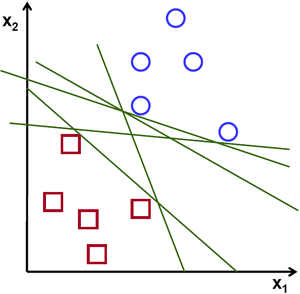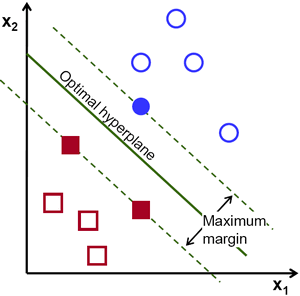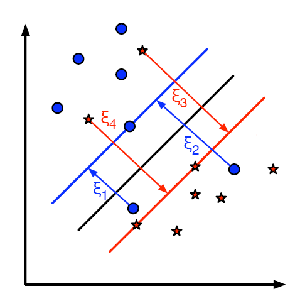# 理解SVM¶

### 理论¶

#### 线性可分数据¶\min_{w, b_0} L(w, b_0) = \frac{1}{2}||w||^2 \; \text{subject to} \; t_i(w^Tx+b_0) \geq 1 \; \forall i

### 非线性可分数据¶

\begin{aligned} K(p,q) = \phi(p).\phi(q) &= \phi(p)^T \phi(q) \\ &= (p_{1}^2,p_{2}^2,\sqrt{2} p_1 p_2).(q_{1}^2,q_{2}^2,\sqrt{2} q_1 q_2) \\ &= p_1 q_1 + p_2 q_2 + 2 p_1 q_1 p_2 q_2 \\ &= (p_1 q_1 + p_2 q_2)^2 \\ \phi(p).\phi(q) &= (p.q)^2 \end{aligned}## 附加资源¶

1. NPTEL notes on Statistical Pattern Recognition, Chapters 25-29.